Importance: Medium ✭✭
 Author(s): Azarija, Jernej Skrekovski, Riste
 Subject: Graph Theory
 Keywords: number of spanning trees, asymptotics
 Posted by: azi on: April 22nd, 2012
Conjecture   Let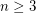be an integer and letdenote the least integersuch that there exists a simple graph onvertices having preciselyspanning trees. Then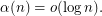Observe thatis well defined forsincehasspanning trees.

The function was introduced by Sedlacek [S] who has shown that for large enoughandUsing the fact that almost all positive integersare expressible as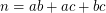for integers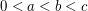it can be shown [A] that for large enoughand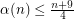otherwise.

Moreover, the only fixed points ofare 3, 4, 5, 6, 7, 10, 13 and 22.

The conjecture is motivated by the following graph (ploted for a very small sample of vertices)The conjecture [C] is justifiable for highly composite numberssince in this case one can construct the graph obtained after taking cycles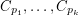for every odd prime factor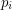of.

## Bibliography

[S] J. Sedlacek, On the minimal graph with a given number of spanning trees, Canad. Math. Bull. 13 (1970) 515-517.

[A] J. Azarija, R. Skrekovski, Euler's idoneal numbers and an inequality concerning minimal graphs with a prescribed number of spanning trees, IMFM preprints 49 (2011) Link to paper

* indicates original appearance(s) of problem.